Anzeige

# Properties of Solids and Liquids Notes - JEE Main 2015

28. Aug 2014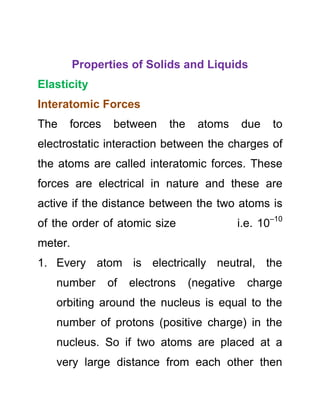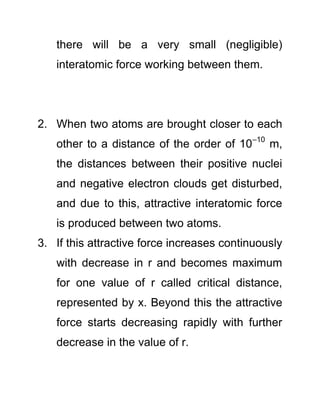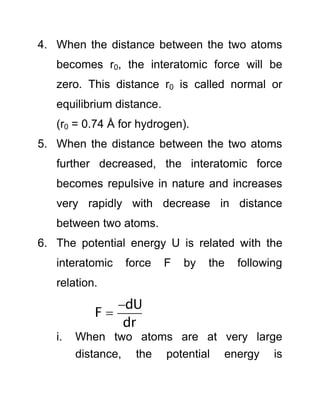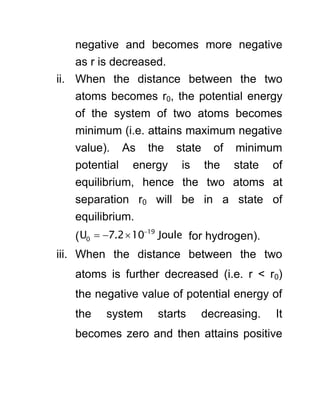Anzeige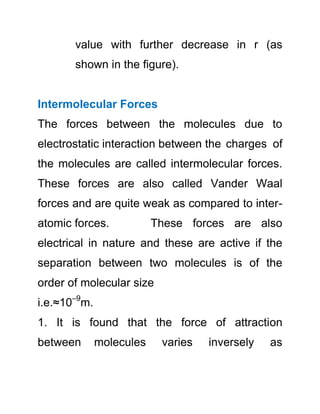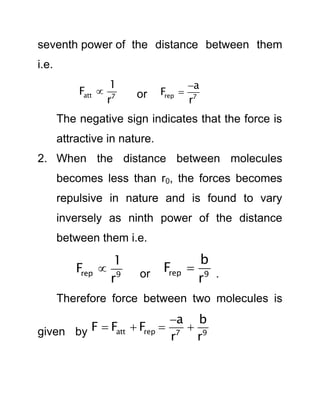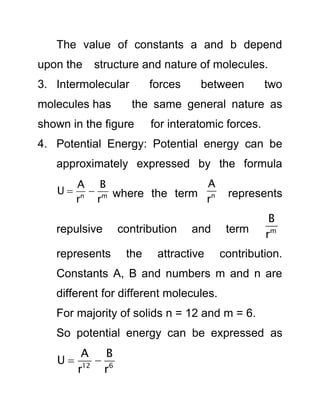Nächste SlideShare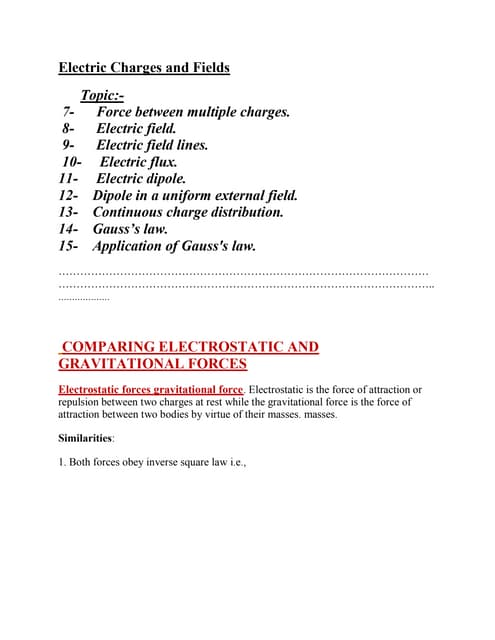Electric charges and fields
1 von 8
Anzeige

### Properties of Solids and Liquids Notes - JEE Main 2015

1. Properties of Solids and Liquids Elasticity Interatomic Forces The forces between the atoms due to electrostatic interaction between the charges of the atoms are called interatomic forces. These forces are electrical in nature and these are active if the distance between the two atoms is of the order of atomic size i.e. 10–10 meter. 1. Every atom is electrically neutral, the number of electrons (negative charge orbiting around the nucleus is equal to the number of protons (positive charge) in the nucleus. So if two atoms are placed at a very large distance from each other then
2. there will be a very small (negligible) interatomic force working between them. 2. When two atoms are brought closer to each other to a distance of the order of 10–10 m, the distances between their positive nuclei and negative electron clouds get disturbed, and due to this, attractive interatomic force is produced between two atoms. 3. If this attractive force increases continuously with decrease in r and becomes maximum for one value of r called critical distance, represented by x. Beyond this the attractive force starts decreasing rapidly with further decrease in the value of r.
3. 4. When the distance between the two atoms becomes r0, the interatomic force will be zero. This distance r0 is called normal or equilibrium distance. (r0 = 0.74 Å for hydrogen). 5. When the distance between the two atoms further decreased, the interatomic force becomes repulsive in nature and increases very rapidly with decrease in distance between two atoms. 6. The potential energy U is related with the interatomic force F by the following relation. dU F dr   i. When two atoms are at very large distance, the potential energy is
4. negative and becomes more negative as r is decreased. ii. When the distance between the two atoms becomes r0, the potential energy of the system of two atoms becomes minimum (i.e. attains maximum negative value). As the state of minimum potential energy is the state of equilibrium, hence the two atoms at separation r0 will be in a state of equilibrium. ( 19 0U 7.2 10 Joule    for hydrogen). iii. When the distance between the two atoms is further decreased (i.e. r < r0) the negative value of potential energy of the system starts decreasing. It becomes zero and then attains positive
5. value with further decrease in r (as shown in the figure). Intermolecular Forces The forces between the molecules due to electrostatic interaction between the charges of the molecules are called intermolecular forces. These forces are also called Vander Waal forces and are quite weak as compared to inter- atomic forces. These forces are also electrical in nature and these are active if the separation between two molecules is of the order of molecular size i.e.≈10–9 m. 1. It is found that the force of attraction between molecules varies inversely as
6. seventh power of the distance between them i.e. att 7 1 F r  or rep 7 a F r   The negative sign indicates that the force is attractive in nature. 2. When the distance between molecules becomes less than r0, the forces becomes repulsive in nature and is found to vary inversely as ninth power of the distance between them i.e. rep 9 1 F r  or rep 9 b F r  . Therefore force between two molecules is given by att rep 7 9 a b F F F r r     
7. The value of constants a and b depend upon the structure and nature of molecules. 3. Intermolecular forces between two molecules has the same general nature as shown in the figure for interatomic forces. 4. Potential Energy: Potential energy can be approximately expressed by the formula n m A B U r r   where the term n A r represents repulsive contribution and term m B r represents the attractive contribution. Constants A, B and numbers m and n are different for different molecules. For majority of solids n = 12 and m = 6. So potential energy can be expressed as 12 6 A B U r r  
8. • Ask Your Doubts • For inquiry and registration, call 9011041155 / 9011031155.
Anzeige